# Number theory

﻿
Number theoryA Lehmer sieve - an analog computer once used for finding primes and solving simple diophantine equations.

Number theory is a branch of pure mathematics devoted primarily to the study of the integers. Number theorists study prime numbers (the building blocks of integers) as well as the properties of objects made out of integers (such as rational numbers) or defined as generalizations of the integers (such as, for example, algebraic integers).

Integers can be considered either in themselves or as solutions to equations (diophantine geometry). Questions in number theory are often best understood through the study of analytical objects (e.g., the Riemann zeta function) that encode properties of the integers, primes or other number-theoretic objects in some fashion (analytic number theory). One may also study real numbers in relation to rational numbers, e.g., as approximated by the latter (diophantine approximation).

The older term for number theory is arithmetic; by the early twentieth century, it had been superseded by "number theory". (The word "arithmetic" is used by the general public to mean "elementary calculations"; it has also acquired other meanings in mathematical logic, as in Peano arithmetic, and computer science, as in floating point arithmetic.) The use of the term arithmetic for number theory regained some ground in the second half of the 20th century, arguably in part due to French influence. In particular, arithmetical is preferred as an adjective to number-theoretic.

## History

### Origins

#### The dawn of arithmetic

The first historical find of an arithmetical nature is a fragment of a table: the broken clay tablet Plimpton 322 (Larsa, Mesopotamia, ca. 1800 BCE) contains a list of "Pythagorean triples", i.e., integers$(a,b,c)$ such that$a^2+b^2=c^2$. The triples are too many and too large to have been obtained by brute force. The heading over the first column reads: "The takiltum of the diagonal which has been substracted such that the width..."

The table's outlay suggests that it was constructed by means of what amounts, in modern language, to the identity$\left(\frac{1}{2} \left(x - \frac{1}{x}\right)\right)^2 + 1 = \left(\frac{1}{2} \left(x + \frac{1}{x}\right)\right)^2,$

which is implicit in routine Old Babylonian exercises. If some other method was used, the triples were first constructed and then reordered by c / a, presumably for actual use as a "table", i.e., with a view to applications.

We do not know what these applications may have been, or whether there could have been any; Babylonian astronomy, for example, truly flowered only later. It has been suggested instead that the table was a source of numerical examples for school problems.

While Babylonian number theory – or what survives of Babylonian mathematics that can be called thus – consists of this single, striking fragment, Babylonian algebra (in the secondary-school sense of "algebra") was exceptionally well developed. Late Neoplatonic sources state that Pythagoras learned mathematics from the Babylonians. (Much earlier sources state that Thales and Pythagoras travelled and studied in Egypt.)

Euclid IX 21—34 is very probably Pythagorean; it is very simple material ("odd times even is odd", "if an odd number measures [= divides] an even number, then it also measures [= divides] half of it"), but it is all that is needed to prove that$\sqrt{2}$ is irrational. (Pythagoraean mystics gave great importance to the odd and the even.) The discovery that$\sqrt{2}$ is irrational is credited to the early Pythagoreans (pre-Theodorus). By revealing (in modern terms) that numbers could be irrational, this discovery seems to have provoked the first foundational crisis in mathematical history; its proof or its divulgation are sometimes credited to Hippasus, who was expelled or split from the Pythagorean sect. It is only here that we can start to speak of a clear, conscious division between numbers (integers and the rationals – the subjects of arithmetic) and lengths (real numbers, whether rational or not).

The Pythagorean tradition spoke also of so-called polygonal or figured numbers. While square numbers, cubic numbers, etc., are seen now as more natural than triangular numbers, square numbers, pentagonal numbers, etc., the study of the sums of triangular and pentagonal numbers would prove fruitful in the early modern period (17th to early 19th century).

We know of no clearly arithmetical material in ancient Egyptian or Vedic sources, though there is some algebra in both. The Chinese remainder theorem appears as an exercise  in Sun Zi's Suan Ching (also known as Sun Tzu's Mathematical Classic; 3rd, 4th or 5th century CE.). (There is one important step glossed over in Sun Zi's solution: it is the problem that was later solved by Āryabhaṭa's kuṭṭaka – see below.)

There is also some numerical mysticism in Chinese mathematics, but, unlike that of the Pythagoreans, it seems to have led nowhere. Like the Pythagoreans' perfect numbers, magic squares have passed from superstition into recreation.

#### Classical Greece and the early Hellenistic period

Aside from a few fragments, the mathematics of Classical Greece is known to us either through the reports of contemporary non-mathematicians or through mathematical works from the early Hellenistic period. In the case of number theory, this means, by and large, Plato and Euclid, respectively.

Plato had a keen interest in mathematics, and distinguished clearly between arithmetic and calculation. (By arithmetic he meant, in part, theorising on number, rather than what arithmetic or number theory have come to mean.) It is through one of Plato's dialogues—namely, Theaetetus – that we know that Theodorus had proven that$\sqrt{3}, \sqrt{5}, \dots, \sqrt{17}$ are irrational. Theaetetus was, like Plato, a disciple of Theodorus's; he worked on distinguishing different kind of inconmensurables, and was thus arguably a pioneer in the study of number systems. (Book X of Euclid's Elements is described by Pappus as being largely based on Theaetetus's work.)

Euclid devoted part of his Elements to prime numbers and divisibility, topics that belong unambiguously to number theory and are basic thereto (Books VII to IX of Euclid's Elements). In particular, he gave an algorithm for computing the greatest common divisor of two numbers (the Euclidean algorithm; Elements, Prop. VII.2) and the first known proof of the infinitude of primes (Elements, Prop. IX.20).

In 1773, Lessing published an epigram he had found in a manuscript during his work as a librarian; it claimed to be a letter sent by Archimedes to Eratosthenes. The epigram proposed what has become known as Archimedes' cattle problem; its solution (absent from the manuscript) requires solving an indeterminate quadratic equation (which reduces to what would later be misnamed Pell's equation). As far as we know, such equations were first successfully treated by the Indian school. It is not known whether Archimedes himself had a method of solution.

#### Diophantus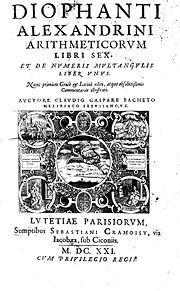Title page of the 1621 edition of Diophantus' Arithmetica, translated into Latin by Claude Gaspard Bachet de Méziriac.

Very little is known about Diophantus of Alexandria; he probably lived in the third century CE, that is, about five hundred years after Euclid. Six out of the thirteen books of Diophantus's Arithmetica survive in the original Greek; four more books survive in an Arabic translation. The Arithmetica is a collection of worked-out problems where the task is invariably to find rational solutions to a system of polynomial equations, usually of the form$f(x,y)=z^2$ or$f(x,y,z)=w^2$. Thus, nowadays, we speak of Diophantine equations when we speak of polynomial equations to which rational or integer solutions must be found.

One may say that Diophantus was studying rational points—i.e., points whose coordinates are rational—on curves and varieties; however, unlike the Greeks of the Classical period, who did what we would now call basic algebra in geometrical terms, Diophantus did what we would now call basic algebraic geometry in purely algebraic terms. In modern language, what Diophantus does is to find rational parametrisations of many varieties; in other words, he shows how to obtain infinitely many rational numbers satisfying a system of equations by giving a procedure that can be made into an algebraic expression (say,$x=g_1(r,s)$,$y=g_2(r,s)$,$z=g_3(r,s)$, where$g_1$,$g_2$ and$g_3$ are polynomials or quotients of polynomials; this would be what is sought for if such$x, y, z$ satisfied a given equation$f(x,y)=z^2$ (say) for all values of r and s).

Diophantus also studies the equations of some non-rational curves, for which no rational parametrisation is possible. He manages to find some rational points on these curves – elliptic curves, as it happens, in what seems to be their first known occurrence—by means of what amounts to a tangent construction: translated into coordinate geometry (which did not exist in Diophantus's time), his method would be visualised as drawing a tangent to a curve at a known rational point, and then finding the other point of intersection of the tangent with the curve; that other point is a new rational point. (Diophantus also resorts to what could be called a special case of a secant construction.)

While Diophantus is concerned largely with rational solutions, he assumes some results on integer numbers; in particular, he seems to assume that every integer is the sum of four squares, though he never states as much explicitly.

#### The Indian school: Āryabhaṭa, Brahmagupta, Bhāskara

While Greek astronomy – thanks to Alexander's conquests – probably influenced Indian learning, to the point of introducing trigonometry, it seems to be the case that Indian mathematics is otherwise an indigenous tradition; in particular, there is no evidence that Euclid's Elements reached India before the 18th century.

Āryabhaṭa (476–550 CE) showed that pairs of simultaneous congruences$n\equiv a_1 \pmod m_1$,$n\equiv a_2 \pmod m_2$ could be solved by a method he called kuṭṭaka, or pulveriser; this is a procedure close to (a generalisation of) the Euclidean algorithm, which was probably discovered independently in India. Āryabhaṭa seems to have had in mind applications to astronomical calculations.

Brahmagupta (628 CE) started the systematic study of indefinite quadratic equations—in particular, the misnamed Pell equation, in which Archimedes may have first been interested, and which did not start to be solved in the West until the time of Fermat and Euler. Later Sanskrit authors would follow, using Brahmagupta's technical terminology. A general procedure (the chakravala, or "cyclic method") for solving Pell's equation was finally found by Jayadeva (cited in the eleventh century; his work is otherwise lost); the earliest surviving exposition appears in Bhāskara II's Bīja-gaṇita (twelfth century).

Unfortunately, Indian mathematics remained largely unknown in the West until the late eighteenth century; Brahmagupta and Bhāskara's work was translated (into English, by Colebrooke) in 1817.

#### Arithmetic in the Islamic golden age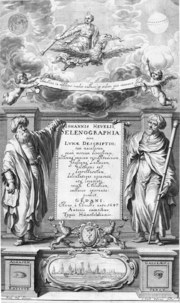Al-Haytham seen by the West: frontispice of Selenographia, showing Alhasen [sic] representing knowledge through reason, and Galileo representing knowledge through the senses.

In the early ninth century, the caliph Al-Ma'mun ordered translations of many Greek mathematical works and at least one Sanskrit work (the Sindhind, which may or may not be Brahmagupta's Brāhmasphuţasiddhānta), thus giving rise to the rich tradition of Islamic mathematics. Diophantus's main work, the Arithmetica, was translated into Arabic by Qusta ibn Luqa (820–912). Part of the treatise al-Fakhri (by al-Karajī, 953 – ca. 1029) builds on it to some extent. Al-Karajī's contemporary Ibn al-Haytham knew what would later be called Wilson's theorem, which, arguably, was thus the first clearly non-trivial result on congruences to prime moduli ever known.

Other than a treatise on squares in arithmetic progression by Fibonacci – who lived and studied in north Africa and Constantinople during his formative years, ca. 1175–1200 – no number theory to speak of was done in western Europe while it went through the Middle Ages. Matters started to change in Europe in the late Rennaissance, thanks to a renewed study of the works of Greek antiquity. A key catalyst was the textual emendation and translation into Latin of Diophantus's Arithmetica (Bachet, 1621, following a first attempt by Xylander, 1575).

### Early modern number theory

#### Fermat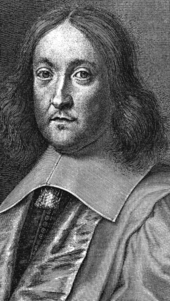Pierre de Fermat

Pierre de Fermat (1601–1665) never published his writings; in particular, his work on number theory is contained entirely in letters to mathematicians and in private marginal notes. He wrote down nearly no proofs in number theory; he had no models in the area. He did make repeated use of mathematical induction, introducing the method of infinite descent.

One of Fermat's first interests was perfect numbers (which appear in Euclid, Elements IX) and amicable numbers; this led him to work on integer divisors, which were from the beginning among the subjects of the correspondence (1636 onwards) that put him in touch with the mathematical community of the day. He had already studied Bachet's edition of Diophantus carefully; by 1643, his interests had shifted largely to diophantine problems and sums of squares (also treated by Diophantus).

Fermat's achievements in arithmetic include:

• Fermat's little theorem (1640), stating that, if a is not divisible by a prime p, then$a^{p-1} \equiv 1 \pmod p.$
• If a and b are coprime, then$a^2 + b^2$ is not divisible by any prime congruent to −1 modulo 4. Every prime congruent to −1 modulo 4 can be written in the form$a^2 + b^2$. These statements date from 1640; in 1659, Fermat stated to Huygens that he had proven the latter statement by the method of descent. Fermat and Frenicle also did some work (some of it erroneous or non-rigorous) on other quadratic forms.
• Fermat posed the problem of solving$x^2 - N y^2 = 1$ as a challenge to English mathematicians (1657). The problem was solved in a few months by Wallis and Brouncker. Fermat considered their solution valid, but pointed out they had provided an algorithm without a proof (as had Jayadeva and Bhaskara, though Fermat would never know this). He states that a proof can be found by descent.
• Fermat developed methods for (doing what in our terms amounts to) finding points on curves of genus 0 and 1. As in Diophantus, there are many special procedures and what amounts to a tangent construction, but no use of a secant construction.
• Fermat states and proves in his correspondence that$x^{4} + y^{4} = z^{4}$ has no non-trivial solutions in the integers. Fermat also mentioned to his correspondents that$x^3 + y^3 = z^3$ has no non-trivial solutions, and that this could be proven by descent. The first known proof is due to Euler (1753; indeed by descent).

Fermat's claim ("Fermat's last theorem") to have shown there are no solutions to$x^n + y^n = z^n$ for all$n\geq 3$ (a fact completely beyond his methods) appears only on his annotations on the margin of his copy of Diophantus; he never claimed this to others and thus had no need to retract it if he found a mistake in his alleged proof.

#### EulerLeonhard Euler

The interest of Leonhard Euler (1707–1783) in number theory was first spurred in 1729, when a friend of his, the amateur Goldbach, pointed him towards some of Fermat's work on the subject. This has been called the "rebirth" of modern number theory, after Fermat's relative lack of success in getting his contemporaries' attention for the subject. Euler's work on number theory includes the following:

• Proofs for Fermat's statements. This includes Fermat's little theorem (generalised by Euler to non-prime moduli); the fact that$p = x^2 + y^2$ if and only if$p\equiv 1\; mod\; 4$; initial work towards a proof that every integer is the sum of four squares (the first complete proof is by Lagrange (1770), soon improved by Euler himself); the lack of non-zero integer solutions to$x^4 + y^4 = z^2$ (implying the case n=4 of Fermat's last theorem, the case n=3 of which Euler also proved by a related method).
• Pell's equation, first misnamed by Euler. He wrote on the link between continued fractions and Pell's equation.
• First steps towards analytic number theory. In his work of sums of four squares, partitions, pentagonal numbers, and the distribution of prime numbers, Euler pioneered the use of what can be seen as analysis (in particular, infinite series) in number theory. Since he lived before the development of complex analysis, most of his work is restricted is restricted to the formal manipulation of power series. He did, however, do some very notable (though not fully rigorous) early work on what would later be called the Riemann zeta function.
• Quadratic forms. Following Fermat's lead, Euler did further research on the question of which primes are can be expressed in the form$x^2 + N y^2$, some of it prefiguring quadratic reciprocity.
• Diophantine equations. Euler worked on some diophantine equations of genus 0 and 1. In particular, he studied Diophantus's work; he tried to systematise it, but the time was not yet ripe for such an endeavour – algebraic geometry was still in its infancy. He did notice there was a connection between diophantine problems and elliptic integrals, whose study he had himself initiated.

#### Lagrange, Legendre and Gauss

Lagrange (1736–1813) was the first to give full proofs of some of Fermat's and Euler's work and observations - for instance, the four-square theorem and the basic theory of the misnamed "Pell's equation" (for which an algorithmic solution was found by Fermat and his contemporaries, and also by Jayadeva and Bhaskara II before them). He also studied quadratic forms in full generality (as opposed to$m X^2 + n Y^2$) -- defining their equivalence relation, showing how to put them in reduced form, etc.

Legendre (1752–1833) was the first to state the law of quadratic reciprocity. He also conjectured what amounts to the prime number theorem and Dirichlet's theorem on arithmetic progressions. He gave a full treatment of the equation$a x^2 + b y^2 + c z^2 = 0$ and worked on quadratic forms along the lines later developed fully by Gauss. In his old age, he was the first to prove "Fermat's last theorem" for n = 5 (completing work by Dirichlet, and crediting both him and Sophie Germain).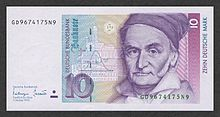Carl Friedrich Gauss

In Disquisitiones Arithmeticae (1798), Gauss (1777–1855) proved the law of quadratic reciprocity and developed the theory of quadratic forms (in particular, defining their composition). He also introduced some basic notation (congruences) and devoted a section to computational matters, including primality tests. The last section of the Disquisitiones established a link between roots of unity and number theory:

The theory of the division of the circle…which is treated in sec. 7 does not belong by itself to arithmetic, but its principles can only be drawn from higher arithmetic.

In this way, Gauss arguably made a first foray towards both Galois's work and algebraic number theory.

### Maturity. Division into subfields.Peter Gustav Lejeune Dirichlet

Starting early in the nineteenth century, the following developments gradually took place:

• The rise to self-consciousness of number theory (or higher arithmetic) as a field of study.
• The development of much of modern mathematics necessary for basic modern number theory: complex analysis, group theory, Galois theory—accompanied by greater rigor in analysis and abstraction in algebra.
• The rough subdivision of number theory into its modern subfields—in particular, analytic and algebraic number theory.

Algebraic number theory may be said to start with the study of reciprocity and cyclotomy, but truly came into its own with the development of abstract algebra and early ideal theory and valuation theory; see below. A conventional starting point for analytic number theory is Dirichlet's theorem on arithmetic progressions (1837), whose proof introduced L-functions and involved some asymptotic analysis and a limiting process on a real variable. The first use of analytic ideas in number theory actually goes back to Euler (1730s), who used formal power series and non-rigourous (or implicit) limiting arguments. The use of complex analysis in number theory comes later: the work of Riemann (1859) on the zeta function is the canonical starting point; Jacobi's four-square theorem (1839), which predates it, belongs to an initially different strand that has by now taken a leading role in analytic number theory (modular forms).

The history of each subfield is briefly addressed in its own section below; see the main article of each subfield for fuller treatments. Many of the most interesting questions in each area remain open and are being actively worked on.

## Main subdivisions

### Elementary tools

The term elementary generally denotes a method that does not use complex analysis. For example, the prime number theorem was first proven in 1896, but an elementary proof was found only in 1949 by Erdős and Selberg. The term is somewhat ambiguous: for example, proofs based on complex Tauberian theorems (e.g. Wiener–Ikehara) are often seen as quite enlightening but not elementary, in spite of using Fourier analysis, rather than complex analysis as such. Here as elsewhere, an elementary proof may be longer and more difficult for most readers than a non-elementary one.

Number theory has the reputation of being a field many of whose results can be stated to the layperson. At the same time, the proofs of these results are not particularly accessible, in part because the range of tools they use is, if anything, unusually broad within mathematics.

### Analytic number theory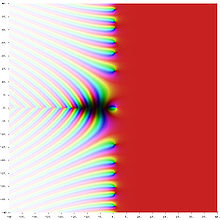Riemann zeta function ζ(s) in the complex plane. The color of a point s gives the value of ζ(s): dark colors denote values close to zero and hue gives the value's argument.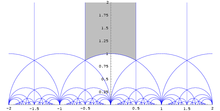The action of the modular group on the upper half plane. The region in grey is the standard fundamental domain.

Analytic number theory may be defined

• in terms of its tools, as the study of the integers by means of tools from real and complex analysis,
• in terms of its concerns, as the study within number theory of estimates on size and density, as opposed to identities.

Some subjects generally considered to be part of analytic number theory, e.g., sieve theory, are better covered by the second rather than the first definition: some of sieve theory, for instance, uses little analysis, yet it is considered to be part of analytic number theory.

The following are examples of problems in analytic number theory: the prime number theorem, the Goldbach conjecture (or the twin prime conjecture, or the Hardy–Littlewood conjectures), the Waring problem and the Riemann Hypothesis. Some of the most important tools of analytic number theory are the circle method, sieve methods and L-functions (or, rather, the study of their properties). The theory of modular forms (and, more generally, automorphic forms) also occupies an increasingly central place in the toolbox of analytic number theory.

One may ask analytic questions about algebraic numbers, and use analytic means to answer such questions; it is thus that algebraic and analytic number theory intersect. For example, one may define prime ideals (generalizations of prime numbers living in the field of algebraic numbers) and ask how many prime ideals there are up to a certain size. This question can be answered by means of an examination of Dedekind zeta functions, which are generalizations of the Riemann zeta function, an all-important analytic object that controls the distribution of prime numbers.

### Algebraic number theory

Algebraic number theory studies algebraic properties and algebraic objects of interest in number theory. (Thus, analytic and algebraic number theory can and do overlap: the former is defined by its methods, the latter by its objects of study.) A key topic is that of the algebraic numbers, which are generalizations of the rational numbers. Briefly, an algebraic number is any complex number that is a solution to some polynomial equation$f(x)=0$ with rational coefficients; for example, every solution x of$x^5 + (11/2) x^3 - 7 x^2 + 9 = 0$ (say) is an algebraic number. Fields of algebraic numbers are also called algebraic number fields, or shortly number fields.

It could be argued that the simplest kind of number fields (viz., quadratic fields) were already studied by Gauss, as the discussion of quadratic forms in Disquisitiones arithmeticae can be restated in terms of ideals and norms in quadratic fields. (A quadratic field consists of all numbers of the form$a + b \sqrt{d}$, where a and b are rational numbers and d is a fixed rational number whose square root is not rational.) For that matter, the 11th-century chakravala method amounts—in modern terms—to an algorithm for finding the units of a real quadratic number field. However, neither Bhāskara nor Gauss knew of number fields as such.

The grounds of the subject as we know it were set in the late nineteenth century, when ideal numbers, the theory of ideals and valuation theory were developed; these are three complementary ways of dealing with the lack of unique factorisation in algebraic number fields. (For example, in the field generated by the rationals and$\sqrt{-5}$, the number 6 can be factorised both as$6 = 2 \cdot 3$ and$6 = (1 + \sqrt{-5}) ( 1 - \sqrt{-5})$; all of 2, 3,$1 + \sqrt{-5}$ and$1 - \sqrt{-5}$ are irreducible, and thus, in a naïve sense, analogous to primes among the integers.) The initial impetus for the development of ideal numbers (by Kummer) seems to have come from the study of higher reciprocity laws, i.e., generalisations of quadratic reciprocity.

Number fields are often studied as extensions of smaller number fields: a field L is said to be an extension of a field K if L contains K. (For example, the complex numbers C are an extension of the reals R, and the reals R are an extension of the rationals Q.) Classifying the possible extensions of a given number field is a difficult and partially open problem. Abelian extensions—that is, extensions L of K such that the Galois group Gal(L/K) of L over K is an abelian group—are relatively well understood. Their classification was the object of the programme of class field theory, which was initiated in the late 19th century (partly by Kronecker and Eisenstein) and carried out largely in 1900—1950.

An example of an active area of research in algebraic number theory is Iwasawa theory. The Langlands program, one of the main current large-scale research plans in mathematics, is sometimes described as an attempt to generalise class field theory to non-abelian extensions of number fields.

### Diophantine geometry

The central problem of Diophantine geometry is to determine when a Diophantine equation has solutions, and if it does, how many. The approach taken is to think of the solutions of an equation as a geometric object.

For example, an equation in two variables defines a curve in the plane. More generally, an equation, or system of equations, in two or more variables defines a curve, a surface or some other such object in n-dimensional space. In Diophantine geometry, one asks whether there are any rational points (points all of whose coordinates are rationals) or integral points (points all of whose coordinates are integers) on the curve or surface. If there are any such points, the next step is to ask how many there are and how they are distributed. A basic question in this direction is: are there finitely or infinitely many rational points on a given curve (or surface)? What about integer points?

An example here may be helpful. Consider the Pythagorean equation x2 + y2 = 1; we would like to study its rational solutions, i.e., its solutions (x,y) such that x and y are both rational. This is the same as asking for all integer solutions to a2 + b2 = c2; any solution to the latter equation gives us a solution x = a / c, y = b / c to the former. It is also the same as asking for all points with rational coordinates on the curve described by x2 + y2 = 1. (This curve happens to be a circle of radius 1 around the origin.)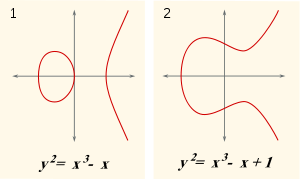Two examples of an elliptic curve, i.e., a curve of genus 1 having at least one rational point. (Either graph can be seen as a slice of a torus in four-dimensional space.)

The rephrasing of questions on equations in terms of points on curves turns out to be felicitous. The finiteness or not of the number of rational or integer points on an algebraic curve—that is, rational or integer solutions to an equation f(x,y) = 0, where f is a polynomial in two variables—turns out to depend crucially on the genus of the curve. The genus can be defined as follows: allow the variables in f(x,y) = 0 to be complex numbers; then f(x,y) = 0 defines a 2-dimensional surface in (projective) 4-dimensional space (since two complex variables can be decomposed into four real variables, i.e., four dimensions). Count the number of (doughnut) holes in the surface; call this number the genus of f(x,y) = 0. Other geometrical notions turn out to be just as crucial.

There is also the closely linked area of diophantine approximations: given a number x, how well can it be approximated by rationals? (We are looking for approximations that are good relative to the amount of space that it takes to write the rational: call a / q (with gcd(a,q) = 1) a good approximation to x if$|x-a/q|<\frac{1}{q^c}$, where c is large.) This question is of special interest if x is an algebraic number. If x cannot be well approximated, then some equations do not have integer or rational solutions. Moreover, several concepts (especially that of height) turn out to be crucial both in diophantine geometry and in the study of diophantine approximations.

Diophantine geometry should not be confused with the geometry of numbers, which is a collection of graphical methods for answering certain questions in algebraic number theory. Arithmetic geometry, on the other hand, is a contemporary term for much the same domain as that covered by the term diophantine geometry. The term arithmetic geometry is arguably used most often when one wishes to emphasise the connections to modern algebraic geometry (as in, for instance, Faltings' theorem) rather than to techniques in diophantine approximations.

## Recent approaches and subfields

The areas below date as such from no earlier than the mid-twentieth century, even if they are based on older material. For example, as is explained below, the matter of algorithms in number theory is very old, in some sense older than the concept of proof; at the same time, the modern study of computability dates only from the 1930s and 1940s, and computational complexity from the 1970s.

### Probabilistic number theory

Take a number at random between one and a million. How likely is it to be prime? This is just another way of asking how many primes there are between one and a million. Very well; ask further: how many prime divisors will it have, on average? How many divisors will it have altogether, and with what likelihood? What is the probability that it have many more or many fewer divisors or prime divisors than the average?

Much of probabilistic number theory can be seen as an important special case of the study of variables that are almost, but not quite, mutually independent. For example, the event that a random integer between one and a million be divisible by two and the event that it be divisible by three are almost independent, but not quite.

It is sometimes said that probabilistic combinatorics uses the fact that whatever happens with probability greater than 0 must happen sometimes; one may say with equal justice that many applications of probabilistic number theory hinge on the fact that whatever is unusual must be rare. If certain algebraic objects (say, rational or integer solutions to certain equations) can be shown to be in the tail of certain sensibly defined distributions, it follows that there must be few of them; this is a very concrete non-probabilistic statement following from a probabilistic one.

At times non-rigorous, probabilistic approach leads to a number of heuristic algorithms and open problems, notably Cramér's conjecture.

### Arithmetic combinatorics

Let A be a set of integers. Consider the set A + A consisting of all sums of two elements of A. Is A + A much larger than A? Barely larger? If A + A is barely larger than A, must A have plenty of arithmetic structure \pmod e.g., does it look like an arithmetic progression?

If we begin from a fairly "thick" infinite set A, does it contain many elements in arithmetic progression: a, a + b, a + 2b, a + 3b,$\ldots$ , a + 10b, say? Should it be possible to write large integers as sums of elements of A?

These questions are characteristic of arithmetic combinatorics. This is a presently coalescing field; it subsumes additive number theory (which concerns itself with certain very specific sets A of arithmetic significance, such as the primes or the squares) and, arguably, some of the geometry of numbers, together with some rapidly developing new material. Its focus on issues of growth and distribution accounts in part for its developing links with ergodic theory, finite group theory, model theory, and other fields. The term additive combinatorics is also used; however, the sets A being studied need not be sets of integers, but rather subsets of non-commutative groups, for which the multiplication symbol, not the addition symbol, is traditionally used; they can also be subsets of rings, in which case the growth of A + A and A·A may be compared.

### Computations in number theory

While the word algorithm goes back only to certain readers of al-Khwārizmī, careful descriptions of methods of solution are older than proofs: such methods (that is, algorithms) are as old as any recognisable mathematics—ancient Egyptian, Babylonian, Vedic, Chinese—whereas proofs appeared only with the Greeks of the classical period. An interesting early case is that of what we now call the Euclidean algorithm. In its basic form (namely, as an algorithm for computing the greatest common divisor) it appears as Proposition 2 of Book VII in Elements, together with a proof of correctness. However, in the form that is often used in number theory (namely, as an algorithm for finding integer solutions to an equation$a x + b y = c$, or, what is the same, for finding the quantities whose existence is assured by the Chinese remainder theorem) it first appears in the works of Āryabhaṭa (5th–6th century CE) as an algorithm called kuṭṭaka ("pulveriser"), without a proof of correctness.

There are two main questions: "can we compute this?" and "can we compute it rapidly?". Anybody can test whether a number is prime or, if it is not, split it into prime factors; doing so rapidly is another matter. We now know fast algorithms for testing primality, but, in spite of much work (both theoretical and practical), no truly fast algorithm for factoring.

The difficulty of a computation can be useful: modern protocols for encrypting messages (e.g., RSA) depend on functions that are known to all, but whose inverses (a) are known only to a chosen few, and (b) would take one too long a time to figure out on one's own. For example, these functions can be such that their inverses can be computed only if certain large integers are factorized. While many difficult computational problems outside number theory are known, most working encryption protocols nowadays are based on the difficulty of a few number-theoretical problems.

On a different note—some things may not be computable at all; in fact, this can be proven in some instances. For instance, in 1970, it was proven that there is no Turing machine which can solve all Diophantine equations (see Hilbert's 10th problem). There are thus some problems in number theory that will never be solved. We even know the shape of some of them, viz., Diophantine equations in nine variables; we simply do not know, and cannot know, which coefficients give us equations for which the following two statements are both true: there are no solutions, and we shall never know that there are no solutions.

## Literature

Two of the most popular introductions to the subject are:

• G. H. Hardy and E. M. Wright, An introduction to the theory of numbers, 6th ed., rev. by D. R. Heath-Brown and J. H. Silverman, Oxford University Press, Oxford, 2008 (first published in 1938).
• I. M. Vinogradov, Elements of Number Theory, Mineola, NY: Dover Publications, 2003, reprint of the 1954 edition.

Hardy and Wright's book is a comprehensive classic, though its clarity sometimes suffers due to the authors' insistence on elementary methods. Vinogradov's main attraction consists in its set of problems, which quickly lead to Vinogradov's own research interests; the text itself is very basic and close to minimal.

Popular choices for a second textbook include:

Wikimedia Foundation. 2010.

### Look at other dictionaries:

• number theory — Math. the study of integers and their relation to one another. Also called theory of numbers. [1910 15] * * * Branch of mathematics concerned with properties of and relations among integers. It is a popular subject among amateur mathematicians… …   Universalium

• number theory — noun The branch of pure mathematics concerned with the properties of integers. Factorization has driven many great discoveries in number theory. See Also: arithmetic, mathematics, natural number, number, prime number, theory …   Wiktionary

• number theory — noun Date: 1864 the study of the properties of integers • number theoretic adjective • number theorist noun …   New Collegiate Dictionary

• number theory — branch of mathematics which deals with the natural numbers; numerology …   English contemporary dictionary

• number theory — num′ber the ory n. math. the study of integers and their relation to one another • Etymology: 1910–15 …   From formal English to slang

• number theory — /ˈnʌmbə θɪəri/ (say numbuh thearree) noun the study of numbers (integers) and of the relations which hold between them …   Australian English dictionary

• number theory — noun : the study of the properties of integers …   Useful english dictionary

• Number Theory, An approach through history from Hammurapi to Legendre — is a classic book on the history of number theory, written by André Weil. The book reviews over three millennia of research on numbers but the key focus is on 19th and 20th century mathematicians. See also List of important publications in… …   Wikipedia

• Number Theory Foundation — The Number Theory Foundation (NTF) is a non profit organization based in the United States which supports research and conferences in the field of number theory. The NTF funds the Selfridge prize which is awarded at the ANTS conferences. External …   Wikipedia

• Number Theory Library — NTL Developer(s) Victor Shoup and others Stable release 5.5.2 / August 14, 2009 Written in C++ Operating system Cross platform …   Wikipedia